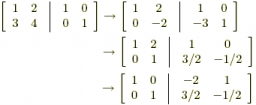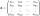# Inverse matrix

Find out inverse by Gauss elimination or by reduction method.
A=[2/3. 1
-3. 1/3]

Correct result:

a =  0.1034
b =  -0.3103
c =  0.931
d =  0.2069

#### Solution:

2/3a+1c = 1
2/3b + 1d = 0
-3a + 1/3c = 0
-3b + 1/3d = 1

2/3•a+1•c = 1
2/3•b + 1•d = 0
-3•a + 1/3•c = 0
-3•b + 1/3•d = 1

2a+3c = 3
2b+3d = 0
9a-c = 0
9b-d = -3

a = 329 ≈ 0.103448
b = -929 ≈ -0.310345
c = 2729 ≈ 0.931034
d = 629 ≈ 0.206897

Our linear equations calculator calculates it.We would be pleased if you find an error in the word problem, spelling mistakes, or inaccuracies and send it to us. Thank you!Tips to related online calculators
Do you have a system of equations and looking for calculator system of linear equations?

## Next similar math problems:

• Inverse matrixFind how many times is the larger determinant is the matrix A, which equals 9 as the determinant of its inverse matrix.
• The inverseThe inverse matrix for matrix A has a determinant value of 0.333. What value has a determinant of the matrix A?
• The determinantThe determinant of the unit matrix equals 7. Check how many rows the A matrix contains.